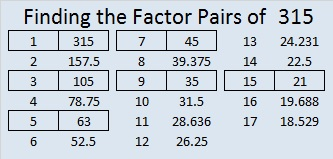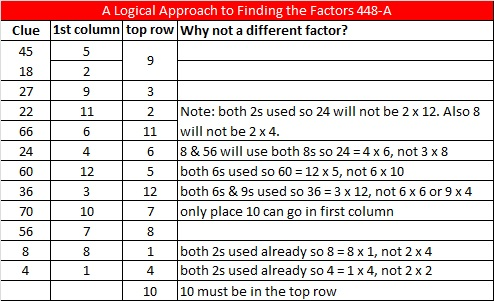# 315 Christmas Factor Trees

315 is the smallest number that is divisible by every 1-digit odd number.

• 315 is a composite number.
• Prime factorization: 315 = 3 × 3 × 5 × 7, which can be written 315 = 3² × 5 × 7
• The exponents in the prime factorization are 2, 1, and 1. Adding one to each and multiplying we get (2 + 1)(1 + 1)(1 + 1) = 3 x 2 x 2 = 12. Therefore 315 has exactly 12 factors.
• Factors of 315: 1, 3, 5, 7, 9, 15, 21, 35, 45, 63, 105, 315
• Factor pairs: 315 = 1 x 315, 3 x 105, 5 x 63, 7 x 45, 9 x 35, or 15 x 21
• Taking the factor pair with the largest square number factor, we get √315 = (√9)(√35) = 3√35 ≈ 17.748Five of the six factor pairs for 315 make lovely Christmas factor trees with the prime factors in red:Here’s a level 4 puzzle that is different kind of Christmas factor tree. Can you complete this mixed-up multiplication table when you’re given only these twelve clues?Print the puzzles or type the factors on this excel file: 12 Factors 2014-12-01